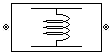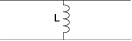# Shunt L

Model shunt inductor

•Libraries:
RF Blockset / Equivalent Baseband / Series / Shunt RLC

## Description

The Shunt L block models the shunt inductor described in the block dialog box, in terms of its frequency-dependent S-parameters

The shunt L object is a two-port network, as shown in the following circuit diagram.## Parameters

expand all

### Main

Scalar value for the inductance. The value must be non-negative.

### Visualization

Frequency data source, specified as `User-specified`.

Frequency data range, specified as a vector in hertz.

Reference impedance, specified as a nonnegative scalar in ohms.

Type of data plot to visualize using the given data, specified as one of the following:

• `X-Y plane` — Generate a Cartesian plot of the data versus frequency. To create linear, semilog, or log-log plots, set the Y-axis scale and X-axis scale accordingly.

• `Composite data` — Plot the composite data. For more information, see Create Plots Using Equivalent Baseband Library Blocks.

• `Polar plane` — Generate a polar plot of the data. The block plots only the range of data corresponding to the specified frequencies.

• `Z smith chart`, ```Y smith chart```, and `ZY smith chart` — Generate a Smith® chart. The block plots only the range of data corresponding to the specified frequencies.

Type of parameters to plot based on the Plot type you set, specified as one of the following.

Plot typeY parameter1
`X-Y plane``S11`, `S12`, `S21`, `S22`, `GroupDelay`, `OIP3`, `NF`, `NFactor`, and `NTemp`.
`Composite data`No Y parameter1 to set.
`Polar plane``S11`, `S12`, `S21`, and `S22`
`Z Smith chart``S11` and `S22`.
`Y Smith chart``S11` and `S22`.
`ZY smith chart``S11` and `S22`.

Type of parameters to plot based on the Plot type you set, specified as one of the following.

Plot typeY parameter2
`X-Y plane``S11`, `S12`, `S21`, `S22`, `GroupDelay`, `OIP3`, `NF`, `NFactor`, and `NTemp`.
`Composite data`No Y parameter2 to set.
`Polar plane``S11`, `S12`, `S21`, and `S22`
`Z Smith chart``S11` and `S22`.
`Y Smith chart``S11` and `S22`.
`ZY smith chart``S11` and `S22`.

Plot format, specified as one of the following.

Y parameter1Y format1
`S11`, `S12`, `S21`, and `S22`.`Magnitude (decibels)`, `Magnitude (linear)`, `Angle(degrees)`, `Angle(radians)`, `Real`, and `Imaginary`.
`GroupDelay``ns`, `us`, `ms`, `s`, and `ps`.
`OIP3``dB`, `dBm`, `W`, and `mW`.
`NF``Magnitude (decibels)`
`NFactor``None`
`NTemp``Kelvin`

#### Dependencies

To enable Y format1, set Plot type to `X-Y plane`.

Plot format, specified as one of the following.

Y parameter2Y format2
`S11`, `S12`, `S21`, and `S22`.`Magnitude (decibels)`, `Magnitude (linear)`, `Angle(degrees)`, `Angle(radians)`, `Real`, and `Imaginary`.
`GroupDelay``ns`, `us`, `ms`, `s`, and `ps`.
`OIP3``dB`, `dBm`, `W`, and `mW`.
`NF``Magnitude (decibels)`
`NFactor``None`
`NTemp``Kelvin`

#### Dependencies

To enable Y format2, set Plot type to `X-Y plane`.

Frequency plot, specified as `Freq`.

Frequency plot format, specified as one of the following.

 `Auto` `Hz` `kHz` `MHz` `GHz` `THz`

Y-axis scale, specified as `Linear` or `Log`.

#### Dependencies

To enable this parameter, set Plot type to ```X-Y plane```.

X-axis scale, specified as `Linear` or `Log`.

#### Dependencies

To enable this parameter, set Plot type to ```X-Y plane```.

Plot the specified data using the plot button.

## Version History

Introduced before R2006a